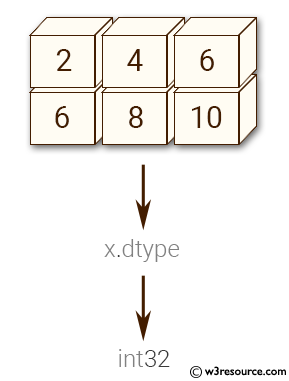﻿ NumPy: Create a 2-dimensional array of size 2 x 3 - w3resource# NumPy: Create a 2-dimensional array of size 2 x 3

## NumPy: Array Object Exercise-37 with Solution

Write a NumPy program to create a 2-dimensional array of size 2 x 3 (composed of 4-byte integer elements), also print the shape, type and data type of the array.

Pictorial Presentation:Sample Solution:-

Python Code:

``````import numpy as np
x = np.array([[2, 4, 6], [6, 8, 10]], np.int32)
print(type(x))
print(x.shape)
print(x.dtype)
```
```

Sample Output:

```<class 'numpy.ndarray'>
(2, 3)
int32
```

Python Code Editor:

Have another way to solve this solution? Contribute your code (and comments) through Disqus.

What is the difficulty level of this exercise?

Test your Python skills with w3resource's quiz

﻿

## Python: Tips of the Day

Set comprehension:

```>>> m = {x ** 2 for x in range(5)}
>>> m
{0, 1, 4, 9, 16}
```